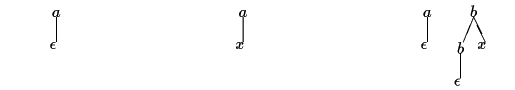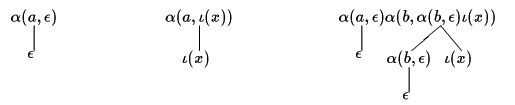# Hedge automata: a formal model for XML schemata

MURATA Makoto (FAMILY Given)

## Introduction

This note shows preliminaries of the hedge automaton theory. In the XML community, this theory has been recently recognized as a simple but powerful model for XML schemata. In particular, the design of RELAX (REgular LAnguage for XML) is directly based on this theory.

## Hedges

First, we introduce hedges. Informally, a hedge is a sequence of trees. In the XML terminology, a hedge is a sequence of elements possibly interevened by character data (or types of character data); in particular, an XML document is a hedge.

A hedge over a finite set Σ (of symbols) and a finite set X (of variables) is:

1. ε (the null hedge),
2. X, where X is a variable in X,
3. a <u>, where a is a symbol in Σ and u is a hedge (the addition of a symbol as the root node), or
4. uv, where u and v are hedges (the concatenation of two hedges).

Figure 1 depicts three hedges: a <ε> , a <x > , and a <ε > b < b <ε> x > . Observe that elements of Σ (i.e., a and b ) are used as labels of non-leaf nodes, while elements of X (i.e., x ) are used as labels of leaf nodes. We abbreviate a <ε> as a. Thus, the third example is denoted by a b <b x > .Figure 1. Three hedges: a <ε> , a <x > , and a <ε > b < b <ε> x >

Next, we consider an XML document. Suppose that Σ = {doc, title, image, para} and X ={#PCDATA}. Then,

doc < title<#PCDATA> para<#PCDATA> <image/> para<#PCDATA>>

is a hedge. In the XML syntax, this hedge can be represented as below:

```  <doc>
<title>#PCDATA</title>
<para>#PCDATA</para>
<image/>
<para>#PCDATA</para>
</doc>
```

## Regular Hedge Grammar

In this section, we introduce regular hedge grammars (RHGs). An RHG is a mechanism for generating hedges. In other words, an RHG describes a set of hedges.

Since the primary role of an XML schema is to describe a set of valid documents, an RHG can be considered as a formal representation of a XML schema.

A regular hedge grammar (RHG) is a 5-tuple <Σ, X, N, P,rf >, where:

• Σ is a finite set of symbols,
• X is a finite set of variables,
• N is a finite set of non-terminals,
• P is a finite set of production rules, each of which takes one of the two forms as below:
• n → x, where n is a non-terminal in N, and x is a variable in X,
• n → a <r>, where n is a non-terminal in N, a is a symbol in Σ, and r is a regular expression comprising non-terminals,
• rf is a regular expression comprising non-terminals.

Now, we consider derivation of RHGs. Informally speaking, given a sequence of non-terminals, we repeatedly replace non-terminals with hedges in the right-hand side of corresponding production rules.

Hedge v is directly derived from hedge u when:

• for some production rule n → x, hedge v is obtained by replacing an occurence of n in u by X, or
• for some production rule n → a <r>, hedge v is obtained by replacing an occurence of n in u by some a <w> such that w is a sequence of non-terminals and matches r.

The language generated by G, denoted by L(G), is the set of hedges which are derived from some non-terminal sequence that matches rf .

Consider an RHG G = < a, x, {n1 , n2}, P, n1?>, where:

P = {n1 → a<n2+ >, n2x }.

Then,

L(G) ={ε, a<x >, a<xx>, a<xxx >, ...}

Next, we construct an RHG that mimicks a DTD. As an example, consider a DTD as follows:

``` <!ELEMENT  doc    (title, (para|image)*)>
<!ELEMENT  title  (#PCDATA)>
<!ELEMENT  para   (#PCDATA)>
<!ELEMENT  image  EMPTY>
```

This DTD can be captured by an RHG G = <Σ, X, N, P, nd > where

• Σ = {doc, title, image, para},
• X = { #PCDATA } ,
• N = {nd , nt , np , ni , n# }
• P = {nddoc <nt (np | ni )* > ,
nttitle <n# >,
nppara <n# >,
niimage <ε>,
n##PCDATA }

Next, consider an RHG G = <Σ, X, N, P, n1 > where:

• Σ = {segment , para} ,
• X = { #PCDATA } ,
• N = { n1 , n2 , np , n# } ,
• P = {n1segment <np* n2* > ,
n2segment <np* > ,
nppara <n# > ,
n##PCDATA }

Both the rule for non-terminal n1 and that for n2 have segment in the right-hand side. However, the former has content model np* n2*, and the latter has content model np*. This impiles that top-level segments can have subordinate segments, but these subordinate segments cannot have subordinate segments.

The DTD syntax cannot exactly capture this RHG, since every occurrence of segments is forced to have the same content model. The smallest DTD that covers this RHG is as below:

```  <!ELEMENT  segment (para*, segment*)>
<!ELEMENT  para    (#PCDATA)>
```

Observe that this DTD allows unlimited nesting of segments. Since the DTD syntax does not allow two content models for segments, this DTD uses one loose content model.

## Hedge Automaton

In this section, we introduce deterministic hedge automata and non-deterministic hedge automata.

A deterministic hedge automaton (DHA) is < Σ, X, Q, α, ι, F>, where:

• Σ is a finite set of symbols,
• X is a finite set of variables,
• Q is a finite set of states,
• α is a function from Σ × Q* to Q such that for every q in Q and x in Σ, {q1 q2 ... qk | k >= 0, α(x, q1 q2 ... qk ) = q } is a regular set,
• ι is a function from X to Q, and
• F is a regular set over Q.

Figure 2 shows the execution of a DHA for a hedge shown in Figure 1.Figure 2.Execution of a deterministic hedge automaton

Next, we show a DHA that accepts the first example in Section 2. Let M = <a , x , { q0, q1, q2 }, α, ι, q1? }, where:

• α(a, u) =
• q1 (u in L(q2+)),
• q0 (otherwise),
• ι(x) = q2 .

Then,

L(G) ={ε , a<x> , a<xx> , a<xxx> , ... }

Next, we introduce non-deterministic hedge automata.

A non-deterministic hedge automaton (NDHA) is < Σ, X, Q, α, ι, F>, where:

• Q, Σ, and F are as specified in the definition of DFA,
• α is a relation (called transition relation) from Σ × Q* to Q (or a function from Σ × Q* to 2Q ) such that for every q in Q and x in Σ, {q0q1...qk | k >= 0, α(x, q0q1...qk, q)} is a regular string language, and
• ι is a relation from X to Q (or a function from X to 2Q).

By definition, a DHA is also a NDHA. We only have to confuse a state and a singleton set containing that state. Thus, the above DHA is also an example of NDHAs.

The last example RHG in Section ``Regular Hedge Grammer'' can be readily captured by a NDHA <Σ, X, Q, α, ι, F >, where

• Σ = {segment, para },
• X = { #PCDATA },
• Q = < q1, q2, qp, q# >,
• α(a, u ) contains q1 when a = segment and u in L ( qp* q2* ) ),
• α(a, u ) contains q2 when a = segment and u in L ( qp* ),
• α(a, u ) contains qp when a = para and u in L(q#)),
• ι(x ) = q# when x = #PCDATA,
• F = q1 .

## Properties of Regular Hedge Languges

### Equivalence

The following conditions are equivalent.

1. L is generated by a RHG,
2. L is accepted by a DHA, and
3. L is accepted by a NDHA.

The proof that (3) implies (2) is done by the subset construction. The rest of the proof is straightforward.

### Boolean closure

Suppose that set L1 and L2 are accepted by (N)DHA M1 and M2, respectively. We can effectively construct (N)DHAs that accept the following languages.

1. the intersection of L1 and L2,
2. the union of L1 and L2,
3. the complement of L1 (the set of all hedges not contained by L1)

### Parse trees of extended context-free grammars

The set of parse trees of an extended context-free grammar is said to be a local tree language. A lot is known about the relationships between local tree languages and regular hedge languages. We mention two observations which are directly relevant to XML.

• A local tree language is a regular hedge language (in other words, for any extenced context-free grammar, we can construct a DHA.), and
• For any regular hedge language that contains trees only, there exists a unique minimal local tree language that includes that regular hedge language.

Observation (1) implies that RHGs are more powerful than DTDs, while (2) ensures that given any RHG, we can construct a reasonable DTD.

### BIBLIOGRAHICS NOTES

In the theoretical computer science community, regular hedge languages were first studied by Pair et al[PQ68] and Takahashi[Tak75]. Regular hedge language can also be considered as extensions of regular tree languages [Tha67]. We borrow some concepts from these papers but adopt definitions more similar to those for regular string languages.

We define RHG's similarily to [PQ68,Tak75], but we avoid projections. Alternatively, our definition can be considered as a hedge-version of Brainerd's tree regular grammars (called "tree generating regular systems) [Bra69].

Our definitions of NDHAs and DHAs are derived from (non-)deterministic tree automata of [Tha67] except that we have extended them to hedges.

It was Kil-Ho Shin (Fuji Xerox) who first proposed to use regular hedge languages as a formal model for schemata of structured documents. His proposal dates back to November, 1991, but he never published any papers. In search of a formalism for document schemata, HIYAMA Masayuki (FAMILY Given) reached a similar formalism in 1996. Since 1993, the present author has applied regular hedge languages (and hedge monoids, which are outside the scope of this note) for schema transformation [Mur97a,Mur97b,Mur98].

The word ``hedge'' was originally proposed by Bruno Courcelle [Cou89]. Derick Wood recommended the use of this word, and it has become the standard word in the XML community after a tutorial by Paul Prescod in 1999. For more information, see the special section on hedge automata in the he SGML/XML Web Page (http://www.oasis-open.org/cover/topics.html#forestAutomata).

### References

[Tha87] James W. Thatcher. Tree automata: An informal survey. In Alfred V. Aho, editor, Currents in the theory of computing, pages 143--172. Prentice-Hall, 1987.